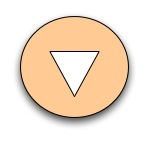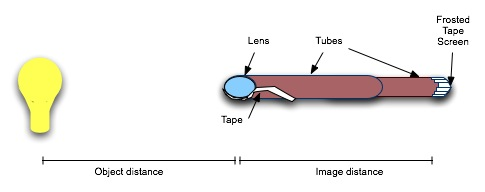Physics Core/AP 1 and 2

#### Course Materials are always under revision! Weblecture content may change anytime prior to two weeks before scheduled chat session for content.Physics Lab

# Physics Lab: Building a small refractor

## Goal: Create a telescope and determine its magnification and resolution

Read about how a telescope focal length is actually determined in practice at Measuring Focal Length.

#### Materials

• Two convex lenses of different diameters and curvatures. (If you have a concave lens you can use, save it for the option below).
• Two cardboard tubes which can be "telescoped" together. If necessary, slit one tube up the side and make it smaller to fit into the other tube.
• Frosted Scotch tape.
• Foam mount. This is a doughnut-shaped piece of foam into which you can fit the lens, then tape the foam in place, so that no tape touches the lens. Foam inserts from packing materials, or the foam "doughnuts" from package of blank DVD/CDs should work.
• Tape measure
• Light source
• Paper and scissors.

#### Procedure

1. Examine your lenses individually, and determine which is the better magnifier. Can you predict this characteristic from the curvature of the lens? How?
2. Trace the diameter of the better magnifier on a piece of paper; cut out the circle, and make a triangular hole in it with the approximate dimensions show below:3. Mount this lens in one end of the larger tube. This is your objective lens.
4. Cover one end of the smaller diameter tube with frosted tape to create a "screen", then insert this tube inside the larger tube as shown below.5. Placing the telescope so the larger lens is at least 1.5m from a light source, measure the distance from the light source to the large lens and record this as the object distance.
6. Adjust the smaller tube so that the image of the light source focuses on the frosted tape "screen", and mark the position of the tube. Measure the distance between the lens and the screen and record this as the image distance.
7. Using the small lens formula 1/di + 1/do = 1/f, determining the focal length 4.
8. Repeat the above measurements with at least three other readings for object distances between 1.5m and 6m (approximated 18 feet).
9. Calculate the average focal length of your larger lens.
10. Find a distance light source (something that can approximate infinity) and adjust for focus. The image distance should equal the focal length.
11. Suppose that you covered the lens with by masking it so that the aperture was smaller. What should happen to your image? (write down your prediction)
12. Now cover the lens with the disk of paper you created in step 2 and record what actually happens to the image.
13. Use a distance source, determine the image distance and focal length of the eyepiece.
14. Remove the frosted paper screen and replace it with your mounted "eyepiece" lens in its foam "case" so that the face of the lens is perpendicular to the face of the objective lens.

Magnification

15. Focus the telescope on a distant object (NOT the sun or other strong light source!).
16. With both eyes open, superimpose the magnified image and the unmagnified image and estimate the amount of magnification. Repeat this until you have a strong sense of the magnifying capability.
17. Using the magnification information, determine the focal length of the eyepiece lens. How does this compare with your distant source determination of the focal length?

Resolution

18. Have someone hold a dime between their fingers as shown below, and back away from you until you see their fingers "touching". Measure the distance to the dime and determine the angle of separation using the width of the dime and the small angle formula α = diameter of dime * 206265/distance to dime.
19. Compare your measured resolution with the predicted resolution θ = 2.5 * 105 λ/D, where D is the diameter of your objective lens. Use 540nm (midrange of visible light) for λ
20. Use your telescope to observe the moon, a distant landscape object. Under what circumstances do you see an upright image? an inverted image?

#### Data Handling

1. Create tables for all of your data.
2. Calculate the focal length of each lens.
3. Calculate the magnification power of your two concave lenses.
4. Calculate the resolution of your telescope and compare with your observations.

#### Report

1. Summarize your data, analysis, calculations, and conclusions.